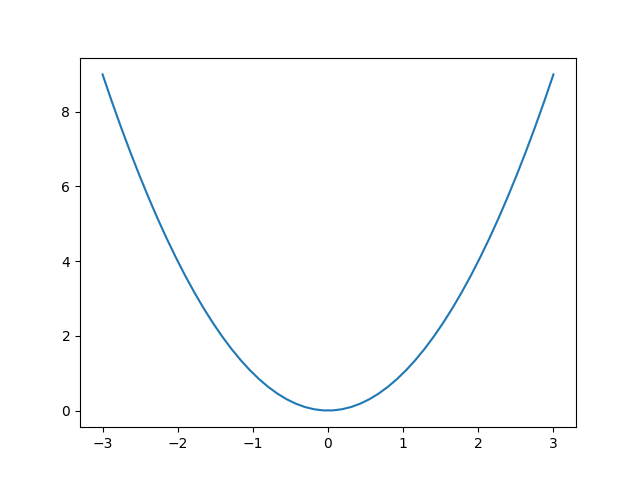# Timers#

Simple example of using general timer objects. This is used to update the time placed in the title of the figure.

Note

This example exercises the interactive capabilities of Matplotlib, and this will not appear in the static documentation. Please run this code on your machine to see the interactivity.

You can copy and paste individual parts, or download the entire example using the link at the bottom of the page.import matplotlib.pyplot as plt
import numpy as np
from datetime import datetime

def update_title(axes):
axes.set_title(datetime.now())
axes.figure.canvas.draw()

fig, ax = plt.subplots()

x = np.linspace(-3, 3)
ax.plot(x, x ** 2)

# Create a new timer object. Set the interval to 100 milliseconds
# (1000 is default) and tell the timer what function should be called.
timer = fig.canvas.new_timer(interval=100)